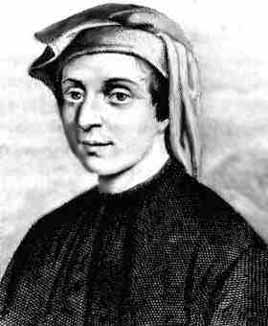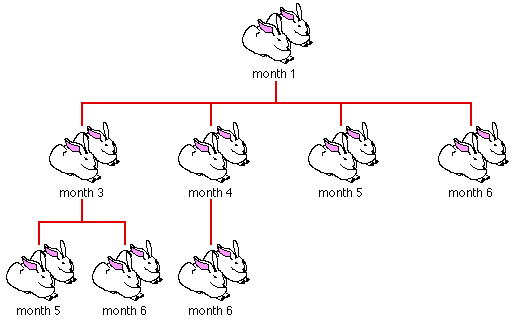FIBONACCI

 Fibonacci (Figure 1) is the name whereby the mathematician Leonardo of Pizza (1170-1250) is commonly known today. In his youth Leonardo was called Filus Bonacci ("son of (Guilielmo) Bonacci"), the name stuck although over the years it has evolved to Fibonacci. He traveled widely, met with scholars through out the Mediterranean area, and produced four very significant works of arithmetic and geometry. One of his discoveries is the sequence of numbers that bears his name --- the Fibonacci sequence. This resulted when, in the early 13th century, Fibonacci posed the following problem:Figure 1: Leanardo Pisano Fibonacci (1170-1250)

A man puts a pair of baby rabbits into an enclosed garden. Assuming each pair of rabbits bears a new pair every month, which from the second month on itself becomes productive, how many pairs of rabbits will there be in the garden after one year.

Like most maths problems realistic happenings such death, escape, impotence, Etc. are ignored. It is not hard to see that the number of pairs of rabbits each month is given by the sequence:

0, 1, 1, 2, 3, 5, 8, 13, 21, 34, 55, 89, 144, 233, 377, ..

After the first two values , 0 and 1, each number of the Fibonacci sequence is obtained from the sum of the preceding two numbers. This corresponds to the fact that each month, the new rabbit births consists of one pair to each of the newly adult pairs plus one pair for each of the earlier adult pairs. This is illustrated in Figure 1.Figure 1: Fibonacci's rabbits

It turns out that the Fibonacci sequence describes all sort of natural phenomena such as: the spiraling pattern on Nautilus shells, elephant tusks, sheep horns, birds claws; the spiral arrangement of seeds on the face of certain sunflower; the pattern on fir cones and pine apples; and the branching patterns many plants and trees. The same number also arises in computer science; for example in database structures, sorting techniques and random number generation.